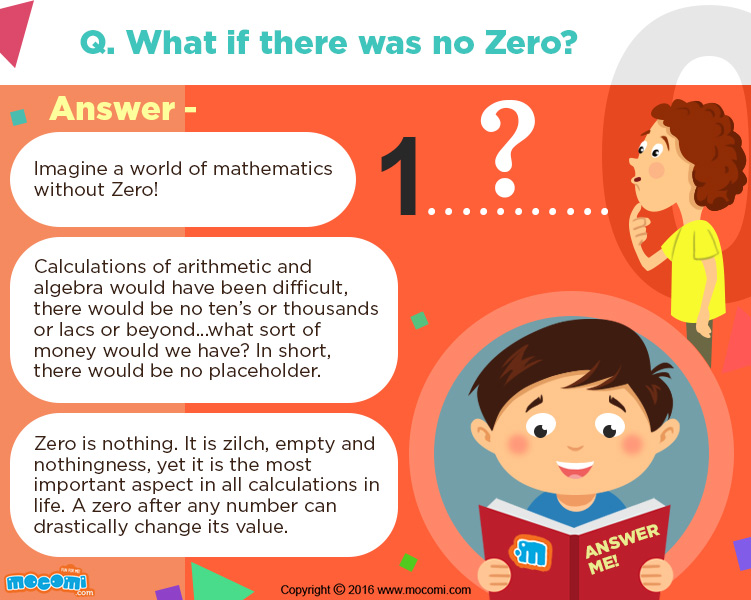• 0
• 0
• 0
• 0
• Embed CodePrevious Article
Next Article

# What if there was no Zero?

### Why do we need Zero?

Zero is the most important number in mathematics. Zero functions as a placeholder. Imagine a number, e.g., 5 and put as many zeroes behind it as you can think of. Zero drastically changes the value of the number from a mere 5 to 50, 500, 5000, 50000 and beyond.

## Origin of the Number Zero?

The concept of zero came from India. We use the Arabic number system today. Prior to the discovery of zero people marked the quantities of items. Every item had a separate symbol and sign. Imagine doing this for every item in today’s world!

It was in India that the concept of zero was first understood. It was understood as both- as a symbol and used in calculations and also as an idea. The idea of zero means that it is nothing; empty!

The Hindu used a small dot called shunya to depict zero. The actual name ‘zero’ was given by the Italians. Europeans resisted using the zero numerical Hindu-Arabic system but later on had to accept it.

## Importance of Zero in Mathematics

Without zero there would be: No algebra, no arithmetic, no decimal, no accounts, no physical quantity to measure, no boundary between negative and positive numbers and most importantly- no computers!